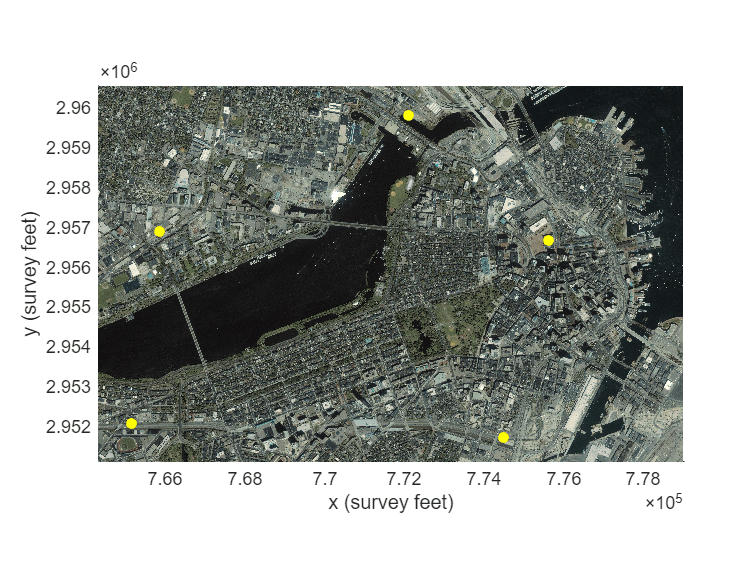# projfwd

Project latitude-longitude coordinates to x-y map coordinates

## Syntax

``````[x,y] = projfwd(proj,lat,lon)``````

## Description

example

``````[x,y] = projfwd(proj,lat,lon)``` transforms the latitude-longitude coordinates specified by `lat` and `lon` to the `x` and `y` map coordinates in the projected coordinate reference system specified by `proj`. Specify `proj` using a `projcrs` object (since R2020b), a map projection structure, or a GeoTIFF information structure.```

## Examples

collapse all

Project latitude-longitude coordinates to x-y coordinates by specifying a map projection. Then, display the projected coordinates on a map.

To do this, first specify the latitude and longitude coordinates of landmarks in Boston. Specify the coordinates in the NAD83 geographic CRS.

```lat = [42.3604 42.3691 42.3469 42.3480 42.3612]; lon = [-71.0580 -71.0710 -71.0623 -71.0968 -71.0941];```

Then, import a GeoTIFF image of Boston as an array and a map cells reference object. Get information about the map projection by querying the `ProjectedCRS` property of the reference object. Verify that the geographic CRS underlying the projected CRS is NAD83.

```[A,R] = readgeoraster('boston.tif'); proj = R.ProjectedCRS; proj.GeographicCRS.Name```
```ans = "NAD83" ```

Project the latitude-longitude coordinates to x-y coordinates using the same projected CRS as the GeoTIFF image.

`[x,y] = projfwd(proj,lat,lon);`

Display the GeoTIFF image and the projected coordinates on the same map. Change the marker symbol and color of the coordinates so they are more visible. Then, add axis labels.

```mapshow(A,R) mapshow(x,y,'DisplayType','point','Marker','o', ... 'MarkerFaceColor','y','MarkerEdgeColor','none') xlabel('x (survey feet)') ylabel('y (survey feet)')```## Input Arguments

collapse all

Map projection, specified as a `projcrs` object (since R2020b), a scalar map projection structure (`mstruct`), or a GeoTIFF information structure. For more information about map projection structures, see `defaultm`. For more information about GeoTIFF information structures, see `geotiffinfo`.

Data Types: `struct`

Geodetic latitudes, specified as a scalar value, vector, matrix, or N-D array, in units of degrees. The size of `lat` and `lon` must match.

Data Types: `single` | `double`

Geodetic longitudes, specified as a scalar value, vector, matrix, or N-D array, in units of degrees. The size of `lat` and `lon` must match.

Data Types: `single` | `double`

## Output Arguments

collapse all

Projected x-coordinates, returned as a scalar value, vector, matrix, or N-D array.

Projected y-coordinates, returned as a scalar value, vector, matrix, or N-D array.

## Tips

If the geographic CRS of `lat` and `lon` does not match the geographic CRS of `proj`, then the values of `x` and `y` may be inaccurate. When `proj` is a `projcrs` object, you can find its geographic CRS by querying its `GeographicCRS` property. For example, this code shows how to create a `projcrs` object from EPSG code 32610 and find the associated geographic CRS.

```proj = projcrs(32610); proj.GeographicCRS.Name```
```ans = "WGS 84"```

## Version History

Introduced before R2006a

expand all